Home > Article index > Circular References

# Working with Circular references in Excel

## Introduction

If you've come to this page, chances are you've experienced the "Circular reference warning" popping up when you opened an Excel file or entered a formula. Excel detects a circular reference when a chain of calculations visits the same cell more than once. Many users get (very) confused by this message and have no idea what it is about. I'll try to demystify that message here!

You may also find an excerpt of parts of the article on the Microsoft Office blog

## Types of circular references

Simplistically speaking there are only a few types of circular references to discern:

### Deliberate circular references

The most important thing to decide up front is whether the model you are trying to calculate actually is of a circular nature. Very often there is a way to rewrite your calculations in such a way that no circular references are needed. This is always the preferred method to use.

Some people deliberately create (sets of) circular reference chains to have a calculation model do iterative calculations. Examples may include models of a chemical facility where (part of) an input stream of a process is a recycled output stream of the same process. Distillation plants often have such streams and if you want to calculate a model of such plant, using a circular reference may be a good way to model your process.

If -and only if- you are convinced you really need circular references to solve your problem, meticulously document your model, stating clearly what your intentions are and how the model functions. Especially important: Devise a way to clearly indicate the circular reference chain.

There are some sub-types of circular references to discern. I list them here under the deliberate circular references, assuming that is the only case where it could be important knowing which subtypes there are.

#### Self-referencing cells

The simplest type of a circular reference is where a cell references itself. This is sometimes used when trying to have a value in a cell which only updates under certain circumstances. An example can be the following:

Suppose you want to keep track of when an entry was made in a certain cell, without using VBA. One way could be the use of the TODAY() function, in combination with the IF function: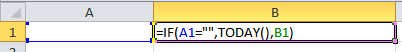Self-referencing cell

As soon as an entry is made in cell A1, the IF function's test evaluates to false, making the formula in cell B1 point to its own value, being the date of the last time Excel recalculated.

#### Single circles

When all cells within a circle are not part of any other circle, we have a single circular reference chain. An example of such a single circle is depicted below: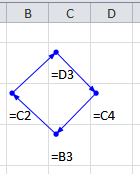A single circular chain of cells

Cell C2 uses the value of cell D3, which uses the value of cell C4, which uses the value of cell B3, which in turn uses the value of the first cell, C2, completing the circle.

#### Multiple circles

To complicate things, cells may be part of more than one circular reference chain. See the example below: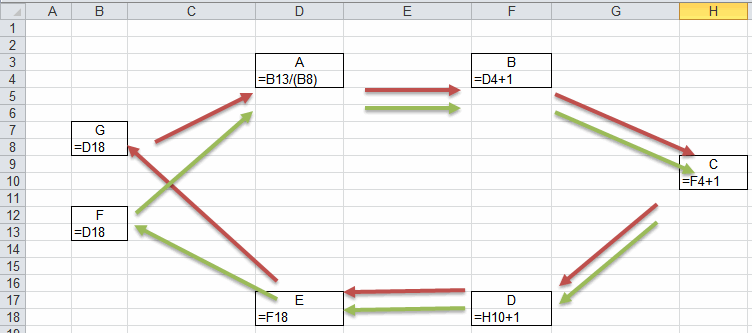Multiple circles

In the example above, there are two circles. The first circle is A-B-C-D-E-F-A (green arrows). The second one is A-B-C-D-E-G-A, the red arrows. Detecting such multiple circular chains is very difficult.

Note that circular reference circles can span multiple worksheets and even multiple workbooks (try to avoid that like the plague!). If you need a circular reference, I advise you to try to keep the cells which are included in the circle as close together as you can so they can be viewed on a single screen all at once. This makes troubleshooting and validating your model easier.

### Accidental circular references

More often, people inadvertently create a circular reference, for example by having a SUM function which includes the cell the SUM function itself resides in.

Example: In cell A10, you write this function: =SUM(A1:A10)

Of course this formula is intended to sum the values in the cells above the cell with the sum function, so the argument for the SUM function should be A1:A9.

## Circular references and calculation settings

If you want to work with circular references, the calculation settings of Excel are very important. This page gives you some pointers!

### Calculation settings

The first thing that needs to be done if you want to assure your model works, is to turn on iterative computation of the file.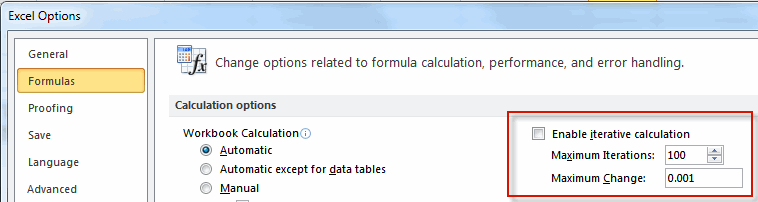Iteration settings in Excel 2010

It is up to you to decide how many iterations you want Excel to do before it stops, or what precision you need before Excel stops (whichever comes first). As soon as you check the box "Enable Iterative calculation", Excel will do a calculation of your model. After saving the file, if you open the file again you should no longer get the circular reference warning message.

If you are troubleshooting your calculation, set Maximum Iterations to 1. This gives you the opportunity to step through the calculations one at the time by repeatedly hitting the F9 key.

### Which calculation settings apply

I often get this question: I have checked the "Enable Iterative calculation" box on my file. Why do I still get the circular reference warning? To be able to understand what causes this it is important to know how Excel handles its calculation settings.

#### Application wide settings

Calculation settings are application-wide. That is, if workbook A needs manual calculation and workbook B needs automatic calculation and you have both workbooks open, Excel's current setting will apply to both workbooks. The same goes for the iterative calculation settings: they apply to all workbooks in your Excel session.

When you save a workbook, whichever calculation setting was applied at that time is saved with the workbook.

#### First-come first-serve

Excel will apply the calculation settings of the first workbook you open in a session. So if you first open workbook A (which had iteration disabled when it was last saved) and then Workbook B (with iteration enabled when it was last saved), Excel will keep iteration disabled. This explains why you do get the circular reference warning on that workbook.

Warning: When you save your workbook, the calculation settings that are currently in effect are saved with the file. This means that if you have previously set up iterative calculation and the max iterations and max change, these settings may be overwritten with the current settings.

### Making sure you have the calculation settings you need

There are several ways to ensure your workbook calculates as expected:

#### Always open as the first workbook

Well, that one is obvious enough. Of course if your model is used by other people as well, this is not exactly fool-proof. Your users would have to be made aware of this situation, but chances are very high your calculation settings will get overwritten at some point, making your model unreliable. And even if you're the sole user, this is a big risk.

#### Use a bit of VBA to control calculation settings

A more reliable way to control the calculation settings is by adjusting them when your workbook loads. This means you will have to add macro's to your file, but this is straightforward enough.

I assume the file with the circular references is already open.

Open its ThisWorkbook module by double-clicking on it in the project explorer as shown below: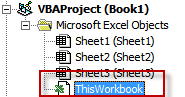The Project explorer in the VBA Editor

Paste this code into the code window that opens up and modify the calculations settings so they match what you need.

Option Explicit

Private Sub Workbook_Open()
With Application
.Calculation = xlCalculationAutomatic
.Iteration = True
.MaxIterations = 100
.MaxChange = 0.001
End With
End Sub

Now save your file (if you are using Excel 2007 or up, make sure you change the file-type to one that can hold macro's, otherwise the macro code is discarded after you close your file!)

## Properly setting up circular references

Whereas I am no fan of using circular references, they can be beneficial to your model and really solve the problem you are trying to solve. So here is some advice on how to properly work with them.

### Breaking the circles and setting initial values

In order to prevent your model from running into trouble, make sure there is a quick way to break each circle. Circular reference chains are risky in that if any of the cells inside a circle inadvertently yields an error value (e.g. a #DIV/0!), your model might not be able to recover and keeps showing error values.

What you can do is have (at least) one cell within each circle which contains an IF function, which -in turn- tests the value of a switch cell. If the switch cell contains anything, the circle is broken and a default value from another cell is taken, instead of the cell that is part of the circular reference chain.

Suppose you created this hypothetic circle: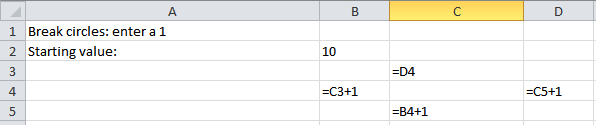A simple circular chain

To get the behavior I just described, I replace the formula in cell C3 with:

=IF(\$B\$1=1,\$B\$2,D4)

Your sheet should now look like this: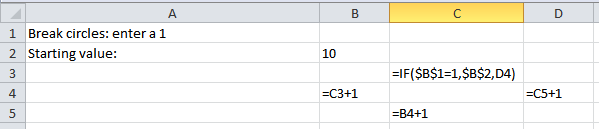A simple circular chain which you can break

Now test your new breakable chain by entering a 1 in cell B1. Cell C3 now will get an initial value of 10 from cell B2. Clear cell B1 to restore the circle.

You can combine the breaker cell with the initial value cell by modifying the formula a bit:

=IF(\$B\$1="",D4,\$B\$1)

This way, you can enter a starting value in cell B1. This breaks the circular chain and sets the starting value. Remove the starting value to start calculating. The disadvantage of combining the break cell and starting value cell is that you loose the starting value as soon as you start the iteration because you have to empty the starting value cell to do so.

A big problem with circular references is that you have to pay attention whether or not your model yields stable results in all circumstances. Iterative calculations can be in these states:

1. The calculation converges

A converging calculation reaches a stable end result, where the last result does not differ more than a fraction from the one-but-last result. Basically, this difference should be less than or equal to the "Maximum Change" setting in your calculation settings.

2. The calculation diverges

When the calculation diverges, things get out of hand: your values keep increasing or decreasing, never to reach a stable end result. The "model" I showed above is an example of a diverging chain. A calculation with results that switch signs (alternating between positive and negative, but with an increasing absolute value) between iterations is also considered to be divergent.

3. The calculation oscillates

The model keeps switching between two (or more) end results.

4. The calculation results do not change, but are incorrect

This is the hardest situation to detect, as you may be under the impression you have reached a solution to your problem. I advise you to find a way to check your results; Are they correct?

So you want to have a converging calculation, I assure you.

One way to check for converging calculation is by setting the maximum iterations to 1. That way you can check the intermediate values by hitting F9, Excel will do one iteration for each press of the F9 key. Pay attention to the results and you'll see whether your calculation converges.

### An alternative approach: have VBA control the circles

An alternative approach to monitor circular reference calculations is by handing over control of the iteration to VBA.

The method I propose here is to break the circles and use intermediate cells which are controlled by VBA to pass on their results to the other cells which used to form the circular reference chain. VBA will perform the iteration and by using some additional cells, the code can closely monitor the results and act accordingly.

For this I took an example from John Walkenbach’s Excel 2010 Bible. A company donates a percentage of its profit after taxes to charity. But since donations to charity are tax-deductible, the donation depends on the tax, which in turn (partly) depends on the donation. A circular reference!

#### Worksheet setup

The figure below shows a spreadsheet with the formulas in place: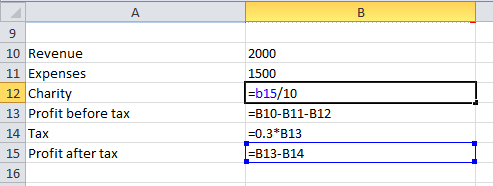The basic principle of the VBA code is that it looks for named ranges in the file which match names like Iter000, Iter001, Iter002, ... The number is unlimited.

To set the model up for the VBA handled iteration these steps are needed.

General settings needed:

• Setup a cell to hold the maximum number of iterations. Name that cell 'Iterations'.
• Have a cell which contains the accuracy and name it Accuracy.
• Have a cell which contains the =NOW() function to make your model volatile so it responds to an F9 key press.

The model changes needed:

• Name any cell Iter### (### being any unique three digit number).
• Name the cell to its immediate right IterResult###.
• Copy a formula from any of the cells within the circular reference chain to this newly named cell. Note that I added 0*[the cell with NOW()] to make the model volatile.
• Change the formula in the cell from which you copied the formula to =IterResult### (### is the number you used when defining the name).
• Have a cell with this formula: =Iter###-IterResult### to get the current delta. Name that cell Delta###.
• Have another cell with a formula like =IFERROR(IF(ABS(Delta###)<Accuracy,1,0),0) and name it IterIsOK###
• Repeat these steps for each circular chain you have.

Now you have broken the circular reference chain. This is what my example looks like, with the formulas in place: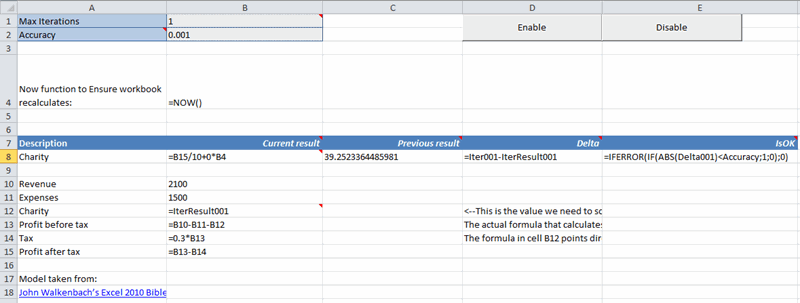The VBA code (see below) takes the Value from the named range Iter### and copies it onto the cell to the immediate right of the named range. The code detects a calculation of Excel and automatically repeats that calculation after copying the Iter### results to their right. It does so the number of times set up in Iterations.

I have also set up a conditional format on range A8:E8: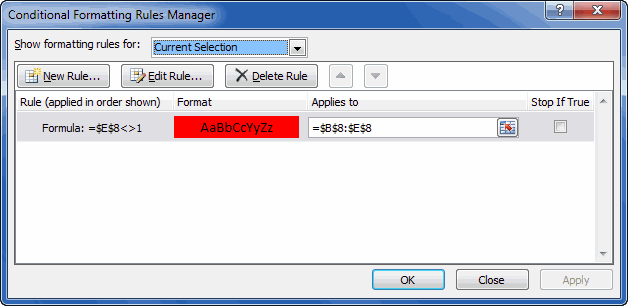The VBA code does not check whether the condition(s) is (are) met, this is handled by the conditional formatting. It is quite easy to update the VBA code to check whether all conditions are met at the end of an iteration and if not, display a warning message.

#### The VBA code

First I'll just list all code. Below you will find an explanation...

'-------------------------------------------------------------------------
' Module    : mIterate
' Company   : JKP Application Development Services (c)
' Author    : Jan Karel Pieterse
' Created   : 10-02-2015
' Purpose   : Handles iteration of a (set of) circular reference(s)
'-------------------------------------------------------------------------
Option Explicit

Dim mlIterations As Long
Dim mlMaxIterations As Long

Const APP_NAME As String = "VBA assisted iteration example"

Public Sub EnableIterations()
Application.OnCalculate = "HandleIterations"
End Sub

Public Sub DisableIterations()
Application.OnCalculate = ""
End Sub

Public Sub HandleIterations()
DisableIterations
mlMaxIterations = ThisWorkbook.Names("Iterations").RefersToRange.Value
If mlIterations >= mlMaxIterations Then
mlIterations = 0
Application.StatusBar = False
EnableIterations
Exit Sub
Else
mlIterations = mlIterations + 1
Application.StatusBar = "Calculating circular references. Iteration # " & mlIterations
CopyIterationValues
Application.Calculate
HandleIterations
End If
EnableIterations
End Sub

Private Function HasIterationFinished() As Boolean
Dim oName As Name
Dim oWs As Worksheet
HasIterationFinished = False
For Each oName In ThisWorkbook.Names
If LCase(oName.Name) Like "iter###" Then
With oName.RefersToRange
If CStr(ThisWorkbook.Names(Replace(oName.Name, "Iter", "IterIsOK")).RefersToRange.Value) = "1" Then
HasIterationFinished = True
Exit Function
End If
End With
End If
Next
End Function

Private Sub CopyIterationValues()
Dim oName As Name
For Each oName In ThisWorkbook.Names
If LCase(oName.Name) Like "iter###" Then
With oName.RefersToRange
On Error Resume Next
ThisWorkbook.Names(Replace(oName.Name, "Iter", "IterResult")).RefersToRange.Value = .Value
On Error GoTo 0
End With
End If
Next
End Sub
##### Entry point routines

The code has two so-called entry point routines, routines called directly by a user action:

EnableIterations and DisableIterations. These two routines are called by the two buttons on the worksheet. They do exactly what their names state.

EnableIterations tells Excel to call HandleIterations after each completion of a calculation.

##### Other routines

HandleIterations first turns off the OnCalculate event by calling DisableIterations. This is done because inside HandleIterations we are asking Excel to recalculate. At that point we do not want HandleIterations to be called again as we want to control when exactly that happens.

For as long as the current number of iterations is less than the maximum number of Iterations, the routine copies the iteration result to its adjacent cell using the routine called CopyIterationValues. If the maximum # of iterations has been reached, the number of iterations is reset to zero and the iteration chain is terminated.

Note that the code also contains a function HasIterationFinished. Currently this function is not used, but you could include the function in HandleIterations to show a message to the user when the iteration has not reached an end result matching your criteria.

## Finding the circular reference chains

Excel does try to help you locate a circular reference. Unfortunately it only shows the first one it encounters in the current calculation chain. You can access that information by Clicking on the Error checking dropdown which is on the Formulas tab of the ribbon: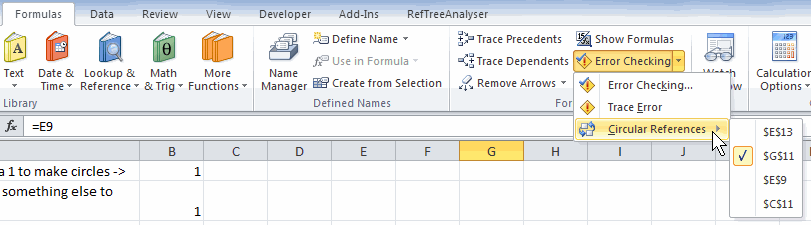If you are looking for a way to view all circular reference chains in the current workbook, I recommend downloading my RefTreeAnalyser utility, it lists all of them in one convenient window: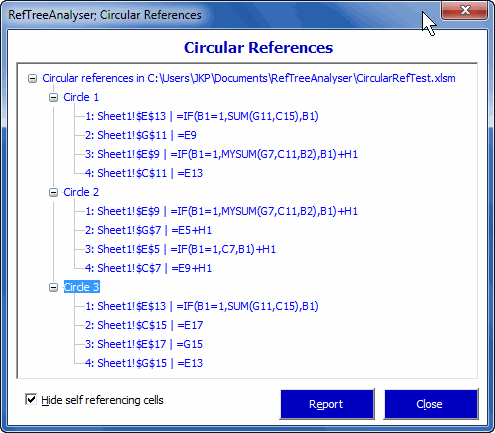## Reasons why circular references may not be detected

### Error results

Sometimes, if cells within a circle have an Error result, the circle may no longer be detected by Excel as being a circular reference (unfortunately, I could not reproduce the problem when I tried to create a demonstration file)

### Values affecting whether a circle is really working as a circle

If one of the cells inside a circle contains a function which may affect which arguments are in use (an IF function, a CHOOSE function, ...), then whether Excel detects a circle depends on that function's proceedings. This is what I used to create switch cells which can break your circles and set initial values.

### Use of arguments in a UDF

Excel is smart. Very smart. Suppose you have a written a VBA User Defined Function (UDF) with two arguments. Excel is smart enough to detect which arguments are actually used in the calculation. So the argument values of the functions used in the circle may affect whether Excel decides it *IS* a circle, because one of the values might cause an argument of a function in the circle not to be used, hence potentially breaking the circle.

### #Name error caused by missing UDF

If any cell within a circle contains a reference to a UDF that isn't available, the circle is not detected.

### Calculation status

When a workbook is opened, calc settings depend on whether or not another workbook is already open in Excel. If you FIRST open a workbook with iteration turned off and THEN open the file with circular references, the circular reference warning shows up. If Iteration is turned on for the first workbook, opening subsequent workbooks does not trigger the warning.